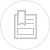# oxidation-reduction

Oxidation-reduction phenomena are very important in every area of water treatment whenever (either as an impurity to be eliminated or as a treatment reagent) elements are involved that are capable of changing the oxidation number (or valency): carbon, nitrogen, sulphur, iron, manganese, chrome, arsenic, chlorine, ozone, etc.

Depending on the experimental conditions, water itself can play a part in oxidation-reduction reactions with the following possible reactions:

In the first case, the water is a donor of electrons; it is a reducing agent: the electrons acceptor is an oxidant and oxygen is formed. In the second case, water is an electrons acceptor; it is an oxidant: the electrons donor is a reducing agent and hydrogen is released.
But without catalysts, the reactions are very slow and, from an oxidation-reduction perspective, generally you can disregard the action water has.

Generally speaking, a weak acid solution’s buffer power is effective for an acid/salt concentrations ratio ranging from 0.1 to 10 and pH = pKa ± 1.

Note: The simultaneous presence of free carbon dioxide and of alkaline or alkaline-earth hydrogen carbonates (bicarbonates) in water provides it with a very useful buffer power, particularly when it is coagulated by a salt with an acid reaction (see typical reagents).

The strength of an oxidation/reduction couple, commonly referred to as a “redox couple”, is defined in relation to the H3O+/H2 reference system (standard or normal hydrogen, ESH or ENH electrode):

Conventionally, the reaction’s standard free enthalpy variation is nil if the hydrogen pressure equals 1 atm and if the [H3O+] ion concentration is 1 mol.L-1.

For an Ox/Red redox couple:

the potential provided by a non-corrodible platinum electrode submerged in a solution at temperature T (K) and which contains both the oxidant and the reducing agents is provided by the Nernst equation:

where F is Faraday’s constant, R is the perfect gases constant, and E° is the standard potential of the Ox/Red couple corresponding to [Ox] = [Red].

The standard potential allows redox reactions to be predicted. For the reaction

due to the interaction of two couples

to be possible, E°1 must be greater than E°2.

Certain redox reactions involve protons or hydroxide ions (for example, the oxidation of Fe2+ by MnO4-). To specify the conditions of these various transformations, you use the potential-pH diagram (or Pourbaix diagram), also known as the stability diagram, because it defines the zones where the various chemical types are thermodynamically stable.

For the general reaction:

at 25°C, the Nernst equation is expressed as:

The potential varies linearly depending on the pH, and the oxidant and reducing agent concentrations. The lines E as a function of the pH, which are drawn for each concentration value, define the thermodynamic zones of the various types of chemicals, which can be solids, gases or in solution. The diagram outlines both the electrochemical and acid-base equilibriums (n=0).

The water diagram was drawn up working from the following respective breakdown and dissociation reactions:

This brings to light (figure 6):

• one oxidation zone I above the line E = 1.23 – 0.06 pH, in which the water breaks down with oxygen being released;
• one reduction zone II below the line E = -0.06 pH, in which the water breaks down with hydrogen being released;
• two oxidation zones Ia and Ib (acid and base respectively) above the equilibrium line for the dissociation reaction (hydrogen partial pressure equal to twice the oxygen partial pressure), E = 0.82 - 0.06.pH , on both sides of the line pH =7;
• two reducing zones IIa and IIb (acid and base respectively), which are below which are below the same chemical equilibrium line, and are separated by the line pH =7.

In zones Ia, Ib, IIa and IIb, the water is thermodynamically stable. Within these zones, only very strong oxidants or reducing agents react with water at a noteworthy speed; for example, chlorine shifts easily to the Cℓ- anion state:

Overall, with water,

with oxygen being released and the medium becoming acidified.

The rH (or rH2) and rO2 index are respectively defined as the decimal logarithm of the reverse of the partial oxygen and hydrogen pressure. The rH index is used to characterise the oxidation state of a system. It can be calculated from the E potential and the pH according to:

where EH is the oxidation-reduction potential compared to the standard hydrogen electrode expressed in Volt.

Note: it will be noted that the foregoing potentials were defined compared to the hydrogen electrode but in fact, the most used electrode on the measuring devices is the electrode known as combined platinum-calomel electrode (Pt-Hg/HgCℓ2)

The following formula makes it possible to go from a potential to an other: